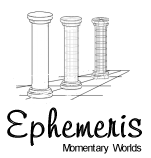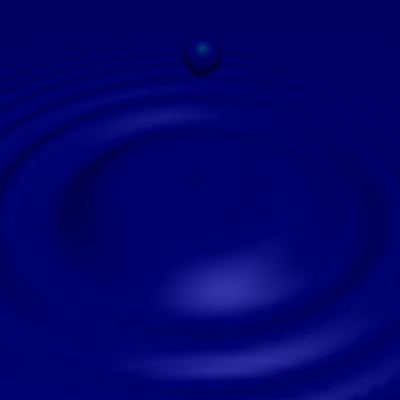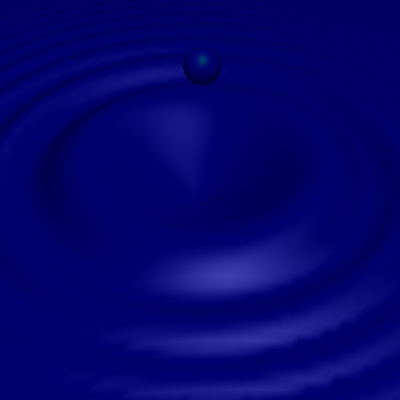Home About Screenshots Download Documentation

Water Droplet SimulationThis is a rendering of the classic water droplet using the AnalyticSurface.  I have included several additional shots as time progresses, since the AnalyticSurface can be dependent on time.  The sphere falls due to the normal effects of gravity.t = 0 sec t = .375 s t = .5 sec t = .575 sec

For those interested in the equation, it is:

f(x, y, z, t) = 0.1 * e -6 Ö(x2+z2) cos[-8t + 36p(x2 + z2) .75 Ö(x2 + z2)]

Because the AnalyticSurface assumes that x, y, z  e  [0, 1] the expression required for the object is offset and scaled a bit.

View the source file for this scene.